# Kawamata rationality theorem

(diff) ← Older revision | Latest revision (diff) | Newer revision → (diff)

A theorem stating that there is a strong restriction for the canonical divisor of an algebraic variety to be negative while the positivity is arbitrary. It is closely related to the structure of the cone of curves and the existence of rational curves.

## Definitions and terminology.

Letbe a normal algebraic variety (cf. Algebraic variety). A-divisor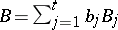onis a formal linear combination of a finite number of prime divisorsofwith rational number coefficients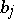(cf. also Divisor). The canonical divisor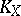is a Weil divisor oncorresponding to a non-zero rational differential-form for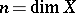(cf. also Differential form). The pairis said to be weakly log-terminal if the following conditions are satisfied:

The coefficients ofsatisfy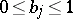.

There exists a positive integersuch that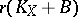is a Cartier divisor (cf. Divisor).

There exists a projective birational morphism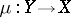from a smooth variety such that the union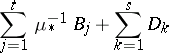is a normal crossing divisor (cf. Divisor), where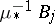is the strict transform ofandcoincides with the smallest closed subsetofsuch that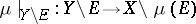is an isomorphism.

One can write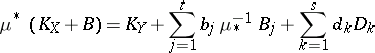such that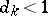for all.

There exist positive integers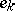such that the divisor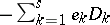is-ample (cf. also Ample vector bundle).

For example, the pair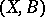is weak log-terminal ifis smooth andis a normal crossing divisor, or ifhas only quotient singularities and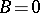.

## Rationality theorem.

Letbe a normal algebraic variety defined over an algebraically closed field of characteristic, and letbe a-divisor onsuch that the pairis weakly log-terminal. Letbe a projective morphism (cf. Projective scheme) to another algebraic variety, and letbe an-ample Cartier divisor on. Then (the rationality theorem, [a1])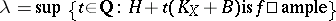is either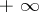or a rational number. In the latter case, letbe the smallest positive integer such thatis a Cartier divisor, and letbe the maximum of the dimensions of geometric fibres of. Express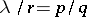for relatively prime positive integersand. Then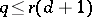.

For example, equality is attained when,,is a point, andis a hyperplane section.

The following theorem asserts the existence of a rational curve, a birational image of the projective line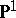, and provides a more geometric picture. However, the estimate of the denominatorobtained is weaker: In the situation of the above rationality theorem, if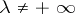, then there exists a morphismsuch that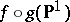is a point and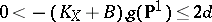[a2].

The two theorems are related in the following way: If, then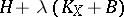is no longer-ample. However, there exists a positive integersuch that the natural homomorphism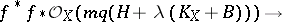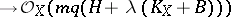is surjective for any positive integer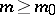(the base-point-free theorem, [a1]). Let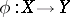be the associated morphism over the base space. Then any positive dimensional fibre ofis covered by a family of rational curves as given in the second theorem [a2].

How to Cite This Entry:
Kawamata rationality theorem. Encyclopedia of Mathematics. URL: http://encyclopediaofmath.org/index.php?title=Kawamata_rationality_theorem&oldid=16450
This article was adapted from an original article by Yujiro Kawamata (originator), which appeared in Encyclopedia of Mathematics - ISBN 1402006098. See original article